Area of Path and Cross roads - Worksheet

Chapter 9 Class 7 Perimeter and Area
Serial order wise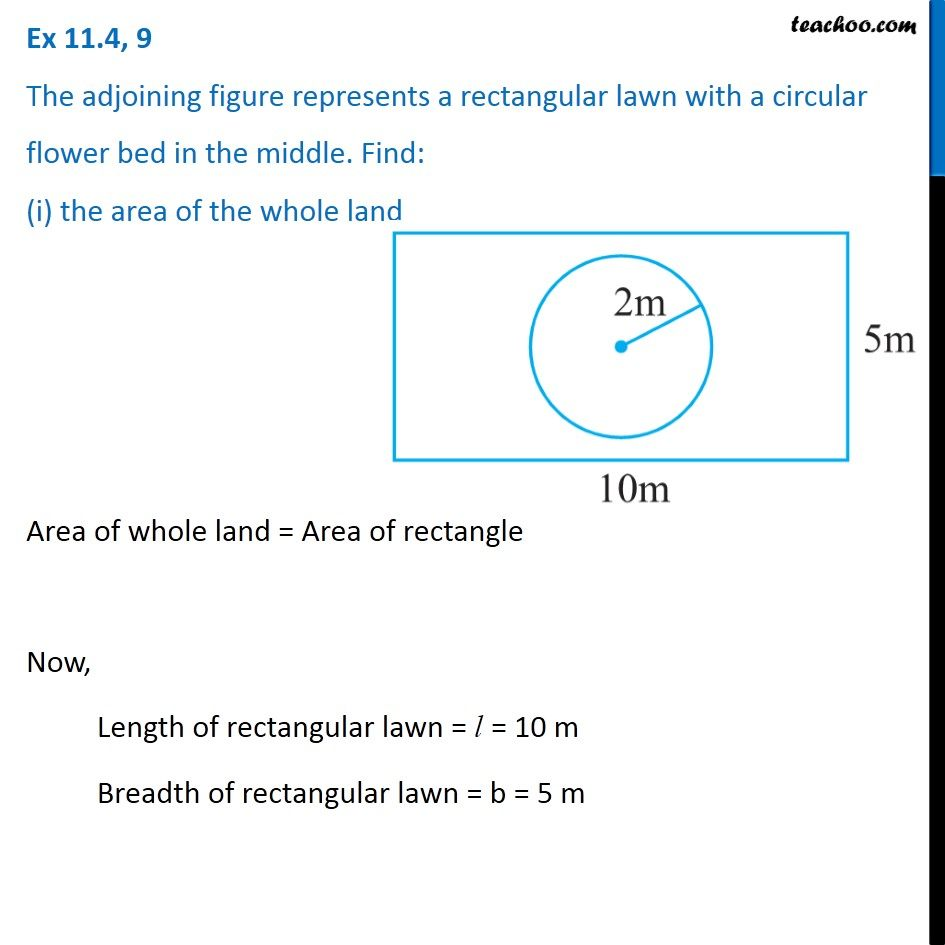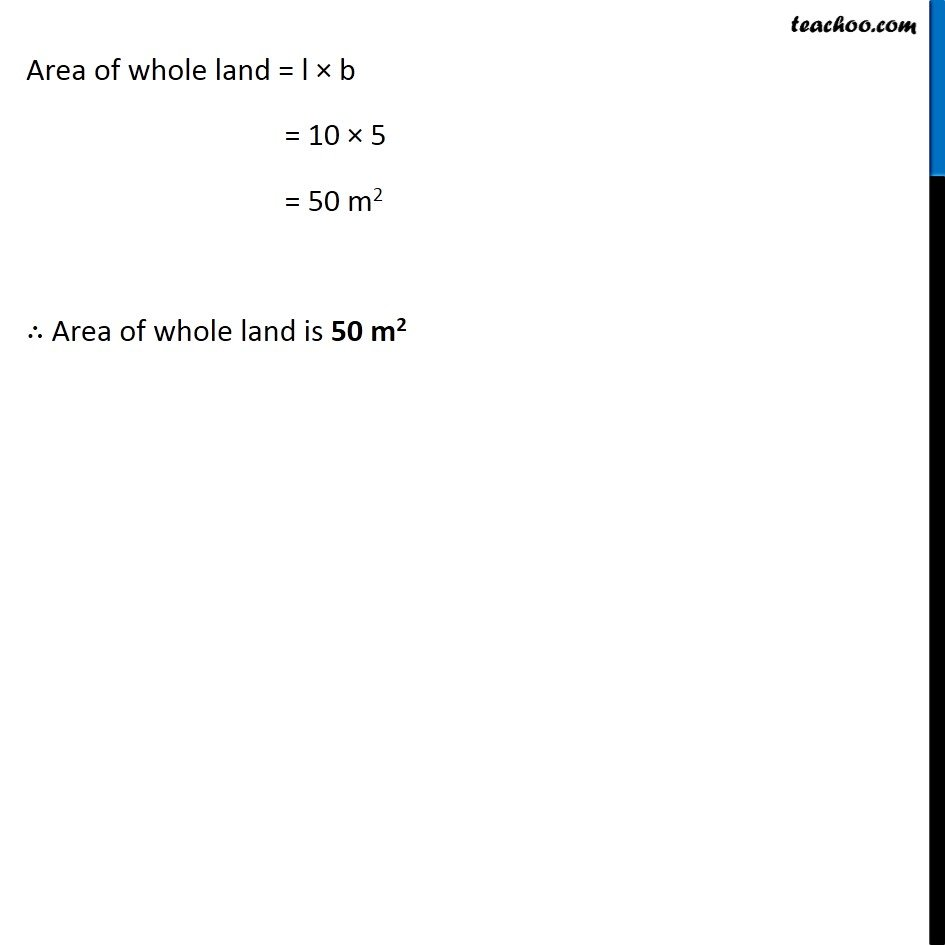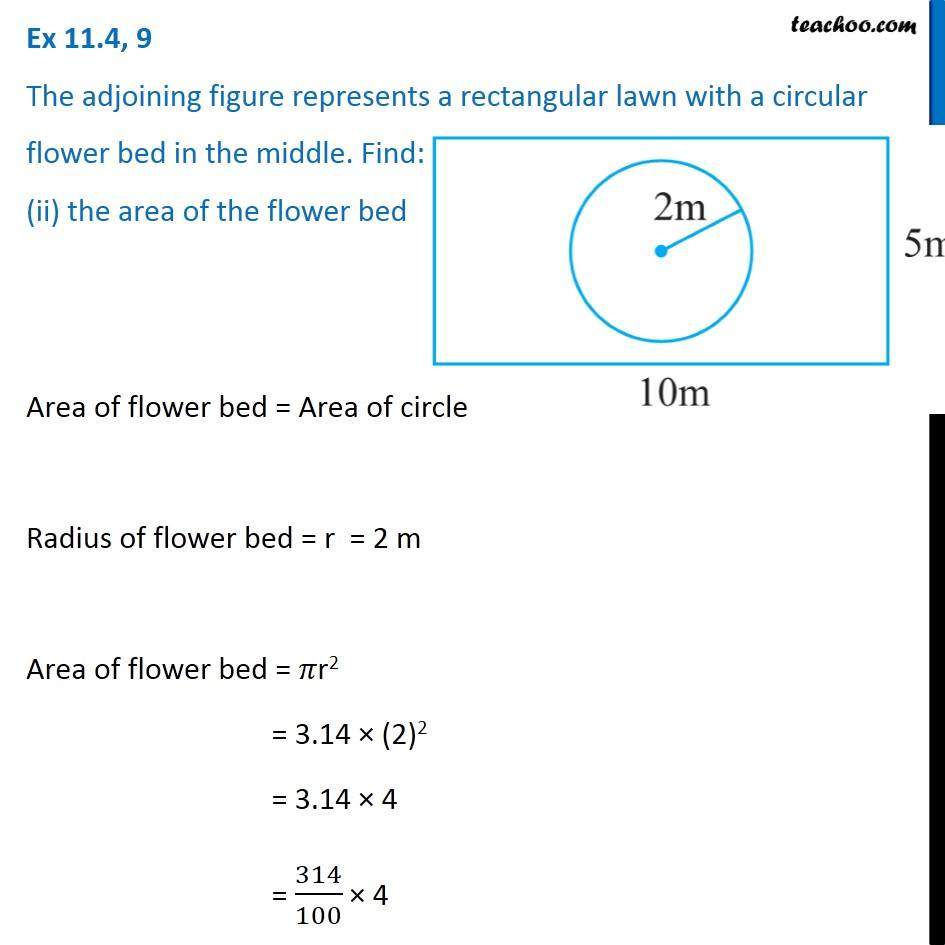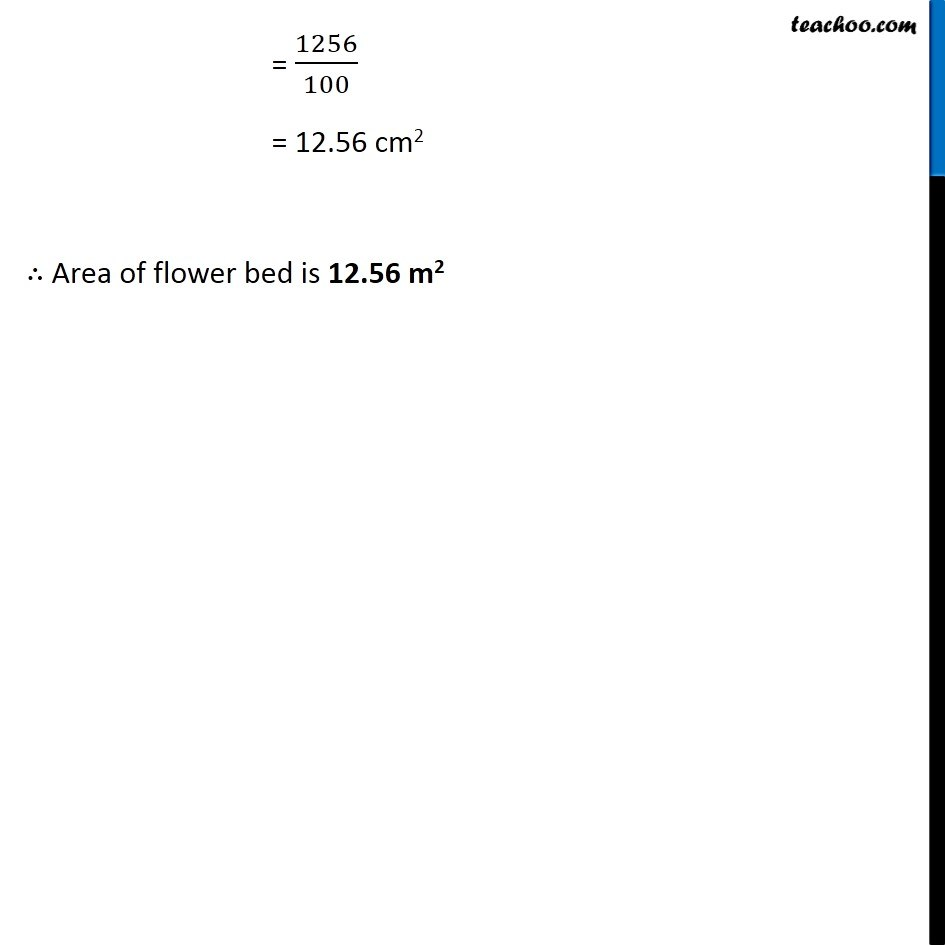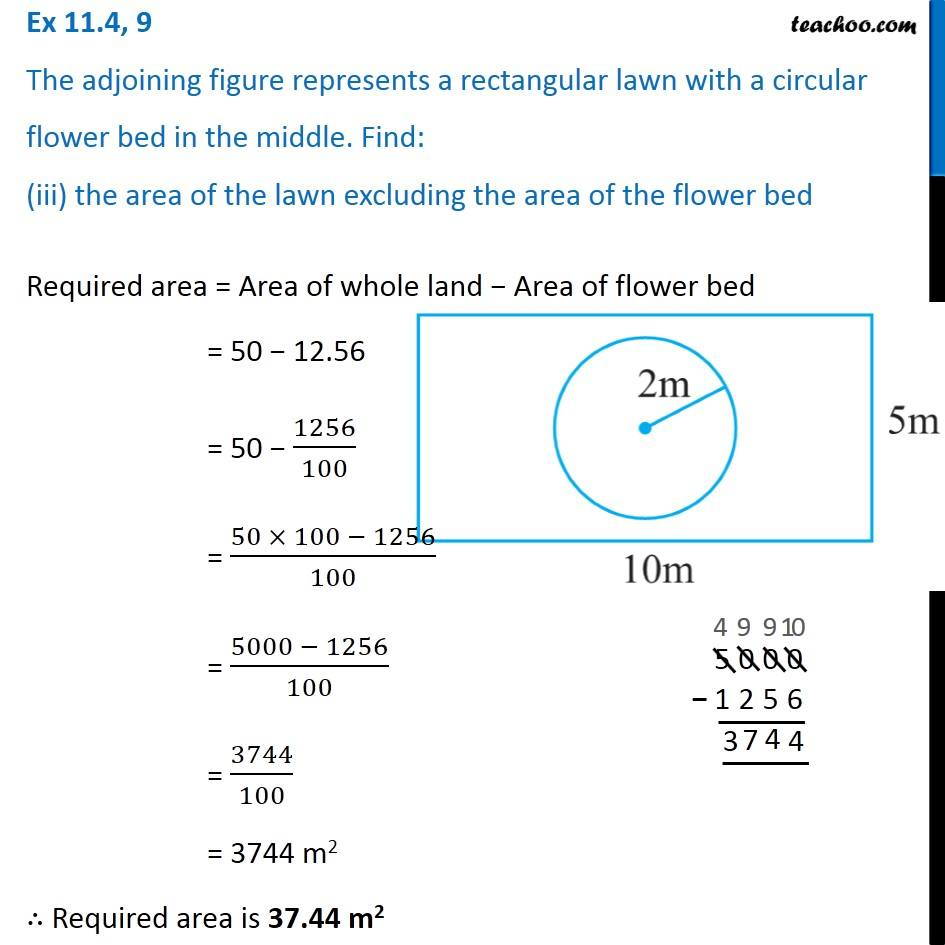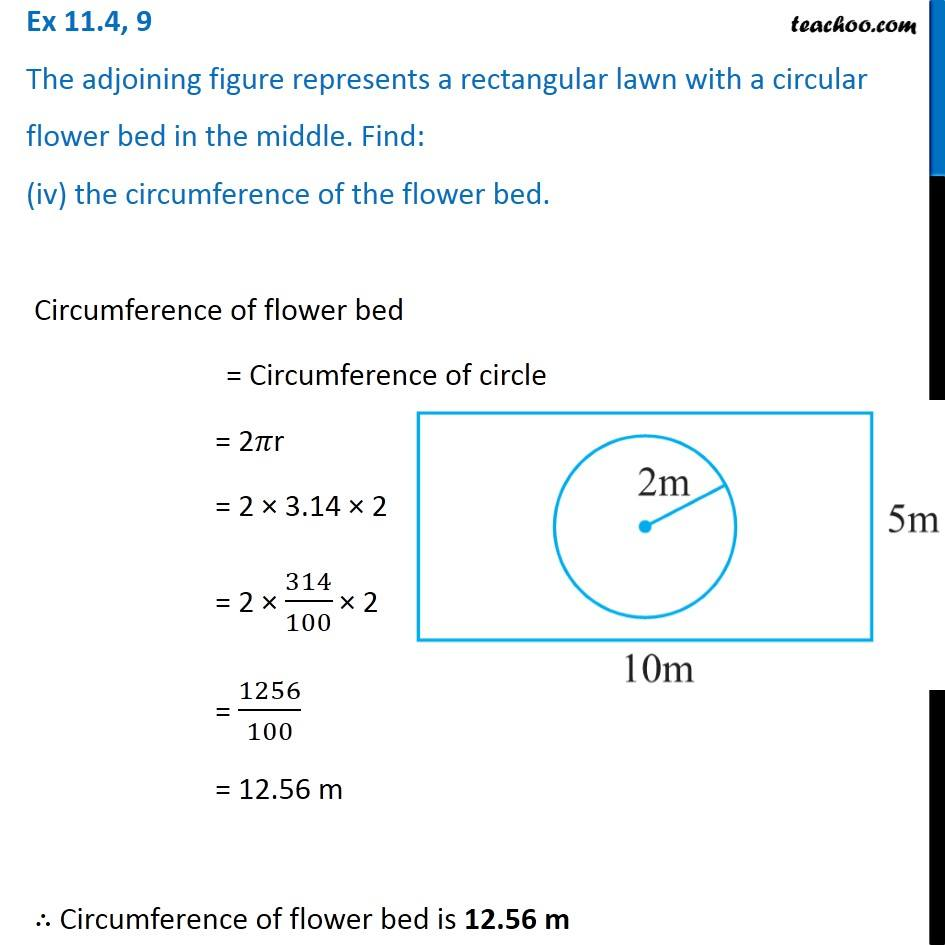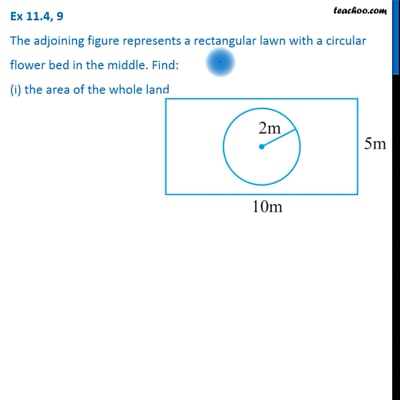This video is only available for Teachoo black users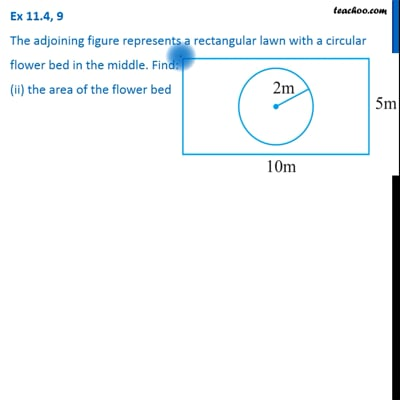This video is only available for Teachoo black users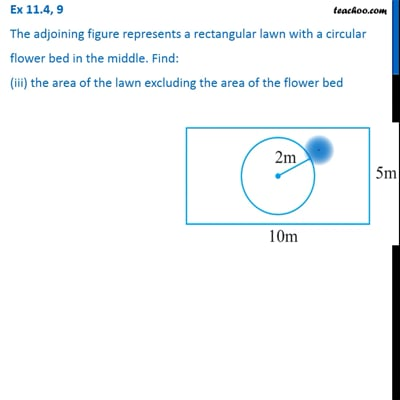This video is only available for Teachoo black users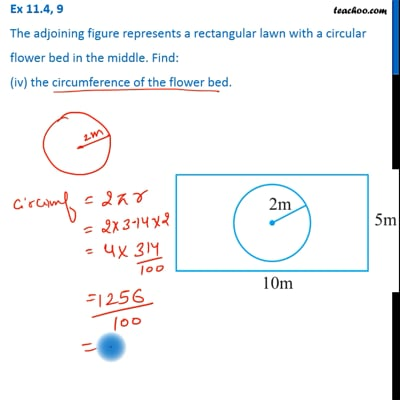This video is only available for Teachoo black users

Learn in your speed, with individual attention - Teachoo Maths 1-on-1 Class

### Transcript

Question 9 The adjoining figure represents a rectangular lawn with a circular flower bed in the middle. Find: (i) the area of the whole land Area of whole land = Area of rectangle Now, Length of rectangular lawn = l = 10 m Breadth of rectangular lawn = b = 5 m Area of whole land = l × b = 10 × 5 = 50 m2 ∴ Area of whole land is 50 m2 Question 9 The adjoining figure represents a rectangular lawn with a circular flower bed in the middle. Find: (ii) the area of the flower bed Area of flower bed = Area of circle Radius of flower bed = r = 2 m Area of flower bed = 𝜋r2 = 3.14 × (2)2 = 3.14 × 4 = 314/100 × 4 = 1256/100 = 12.56 cm2 ∴ Area of flower bed is 12.56 m2 Question 9 The adjoining figure represents a rectangular lawn with a circular flower bed in the middle. Find: (iii) the area of the lawn excluding the area of the flower bed Required area = Area of whole land − Area of flower bed = 50 − 12.56 = 50 − 1256/100 = (50 × 100 − 1256)/100 = (5000 − 1256)/100 = 3744/100 = 3744 m2 ∴ Required area is 37.44 m2 Question 9 The adjoining figure represents a rectangular lawn with a circular flower bed in the middle. Find: (iv) the circumference of the flower bed. Circumference of flower bed = Circumference of circle = 2𝜋r = 2 × 3.14 × 2 = 2 × 314/100 × 2 = 1256/100 = 12.56 m ∴ Circumference of flower bed is 12.56 m